# Spherical Aberration

For lenses made with spherical surfaces, rays which are parallel to the optic axis but at different distances from the optic axis fail to converge to the same point. For a single lens, spherical aberration can be minimized by bending the lens into its best form. For multiple lenses, spherical aberrations can be canceled by overcorrecting some elements. The use of symmetric doublets like the orthoscopic doublet greatly reduces spherical aberration.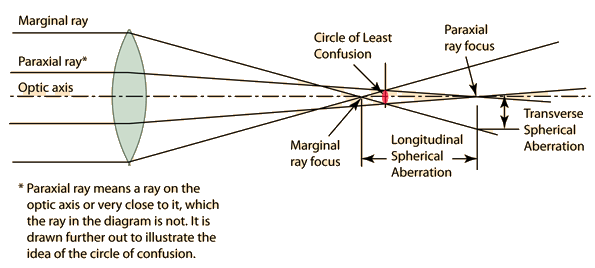When the concept of principal focal length is used, the presumption is that all parallel rays focus at the same distance, which is of course true only if there are no aberrations. The use of the lens equation likewise presumes an ideal lens, and that equation is practically true only for the rays close to the optic axis, the so-called paraxial rays. For a lens with spherical aberration, the best approximation to use for the focal length is the distance at which the difference between the paraxial and marginal rays is the smallest. It is not perfect, but the departure from perfect focus forms what is called the "circle of least confusion". Spherical aberration is one of the reasons why a smaller aperture (larger f-number) on a camera lens will give a sharper image and greater depth of field since the difference between the paraxial and marginal rays is less.

 Lens Aberrations
Index

Lens concepts

Thick lens concepts

 HyperPhysics***** Light and Vision R Nave
Go Back

# Meniscus Lenses

The amount of spherical aberration in a lens made from spherical surfaces depends upon its shape. Bending the lens can also give partial correction of coma aberration.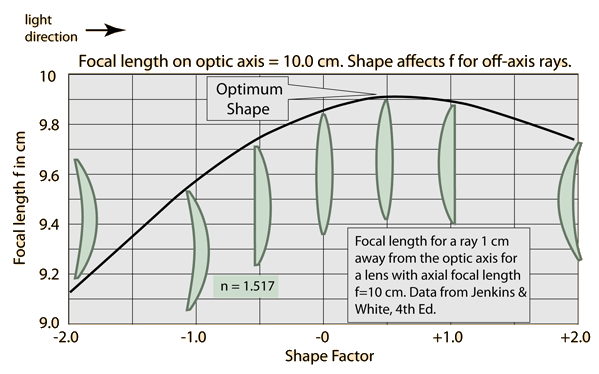Lens Aberrations Minimizing spherical aberration with shape
Index

Lens concepts

"Reference
Jenkins & White
p 156 ff

 HyperPhysics***** Light and Vision R Nave
Go Back

# Coddington Shape Factor

Although spherical aberration cannot be eliminated for a single lens, it can be minimized by the appropriate bending of the lens into its best form. The degree of bending can be characterized by the Coddington shape factor: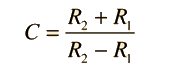Coddington Shape Factor

R1 and R2 are the surface radii of the spherical lens surfaces.

The minimum spherical aberration also depends on the object and image distances, so another factor enters, called the Coddington position factor: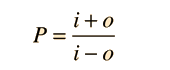Coddington Position Factor

i and o are the image and object distances for the lens as in the lens equation.

The minimum spherical aberration occurs when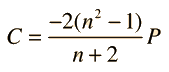Condition for minimum spherical aberration

The higher the index of refraction n, the smaller the aberration for the optimum shape.

 Lens Aberrations
Index

Lens concepts

"Reference
Meyer-Arendt
Ch 5

 HyperPhysics***** Light and Vision R Nave
Go Back